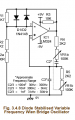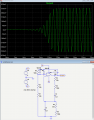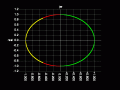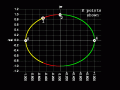# Questions About Variable Frequency Wien Bridge Oscillator

#### electriz

Joined Nov 17, 2020
3
I am trying to design a wein bridge oscillator with variable frequency. I am following a circuit given from this website: https://learnabout-electronics.org/Oscillators/osc34.php

I am running into trouble with the simulations since it doesn't seem to oscillate. I have attached my LT spice file along with this question. Also how could I increase the bandwidth in the Khz range? Thanks in advance#### Attachments

• 2.5 KB Views: 8

#### LvW

Joined Jun 13, 2013
1,669
The resistors as shown in the document give a gain of 3.04 only.
Choose a gain of 3.1----3.2 and give on of the capacitors an initial condition (voltage) for a safe start of oscillation.

Last edited:

#### crutschow

Joined Mar 14, 2008
32,861
I corrected errors in the capacitor values (they both should be the same value) and changed the values of the left resistors (the values are cut off in you schematic) to get it to oscillate (below).
Do you mean the frequency of oscillation?
For that you reduce the capacitor size and/or reduce the right resistor sizes.•electriz

#### Audioguru again

Joined Oct 21, 2019
6,155
An LM324 opamp was designed to use a low power supply current causing it be noisy, produce crossover distortion and have trouble with frequencies above 2kHz.

Modern audio opamps have none of these problems and work well up to at least 100kHz.

The diodes in that circuit cause distortion. My Wien bridge oscillator uses a Jfet for low distortion amplitude control.

#### Audioguru again

Joined Oct 21, 2019
6,155
Crutschow, why does your LTspice produce a stepped sinewave output? Mine doesn't.

#### ericgibbs

Joined Jan 29, 2010
17,992
hi agu,
I guess this was selected.
ref image.
E

#### Audioguru again

Joined Oct 21, 2019
6,155
I do not know which opamp was used. I used a real opamp and the sinewave started up much faster than the other one so my sinewave was not crammed horizontally then my sinewave has many more hard to see steps.

#### electriz

Joined Nov 17, 2020
3
I corrected errors in the capacitor values (they both should be the same value) and changed the values of the left resistors (the values are cut off in you schematic) to get it to oscillate (below).
Do you mean the frequency of oscillation?
For that you reduce the capacitor size and/or reduce the right resistor sizes.

View attachment 222502
Yeah like for example if I wanted the circuit to vary from 1Khz to 100Khz by varying the resistance? Would I change R5 and R6 by the same amount? And also how would the amplitude be varied?

#### Audioguru again

Joined Oct 21, 2019
6,155
Dual potentiometers do not match very well.
My Wien bridge oscillator uses LM07x opamps that have a very high input impedance then the frequency is varied with a variable dual capacitor from an old AM radio that can be adjusted to match perfectly. Vary the amplitude with a volume control at the output,

•electriz

#### MrAl

Joined Jun 17, 2014
10,595
Here is a root locus plot.

The yellow line is when K is varied from 0 to 1.
The red line is when K is varied from 1 to 2.
The green line is when K is varied from 2 to 4.
K is the multiplier of R1+R7 which is then the value of R8:
R8=K*(R1+R7)
For example, if R1+R7=10k then R8=K*10k so where the green line meets the red line K=2 so then R8 would equal 20k. That happens to be the point of perfect oscillation. That means K must be greater than 2 (such as 2.1, 2.2, etc.) but should not be too much higher than 2. Values higher than 2 cause an exponential rise in the sinusoid which left unchecked would saturate the output. The diodes are present to automatically keep K to a value around 2.
Limitations in the op amp will mean K may have to be even higher though because of the limited gain of the imperfect op amp at the chosen oscillation frequency. The exact value of k=2 is derived from using an op amp with infinite gain and zero internal phase shift as usual.Last edited:
•electriz

#### crutschow

Joined Mar 14, 2008
32,861
I wanted the circuit to vary from 1Khz to 100Khz by varying the resistance?
Yes, you can.
I change R5 and R6 by the same amount?
Yes.
And also how would the amplitude be varied?
Add a pot on the output or, if you want a low impedance output, an op amp with a variable gain.
Dual potentiometers do not match very well.
True, but I'm not sure how significant that is in this application.
They don't have to be exactly the same for the oscillator to work.
It may just make the oscillator frequency somewhat non-linear with respect to pot rotation.

Last edited:

#### electriz

Joined Nov 17, 2020
3
Dual potentiometers do not match very well.
My Wien bridge oscillator uses LM07x opamps that have a very high input impedance then the frequency is varied with a variable dual capacitor from an old AM radio that can be adjusted to match perfectly. Vary the amplitude with a volume control at the output,
Where can I find your circuit? Can I take a look?

#### Audioguru again

Joined Oct 21, 2019
6,155
Where can I find your circuit? Can I take a look?
Google is full of it, here:

#### MrAl

Joined Jun 17, 2014
10,595
Google is full of it, here:
That is the most basic theoretical circuit. Not exactly wrong theoretically just not practical especially with R1 and R2 values not shown.

#### LvW

Joined Jun 13, 2013
1,669
Where can I find your circuit? Can I take a look?
Are you aware that there are, of course, oscillator circuits which can be tuned by variation of a single resistor only?
However, in most cases, two opamps are required.

#### MrAl

Joined Jun 17, 2014
10,595
Hi,

Here is the root locus again this time i labeled the K points. I meant to do that in the software but did not get around to that yet.As shown when K=2 we get perfect oscillation at least in the theoretically ideal case.
When K=0 we get a falling exponential with no oscillatory part.
With K=1 we get a falling exponential with some oscillatory part but it damps out over time to zero.
When K=4 we get a rising exponential with no oscillatory part and the output saturates.
When K=3 (somewhere between K=2 and K=4 on the green line) we get a rising exponential with an oscillatory part but the rising exponential again causes the output to saturate.